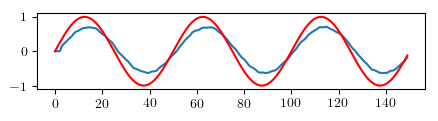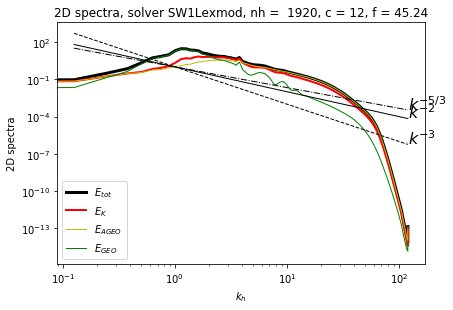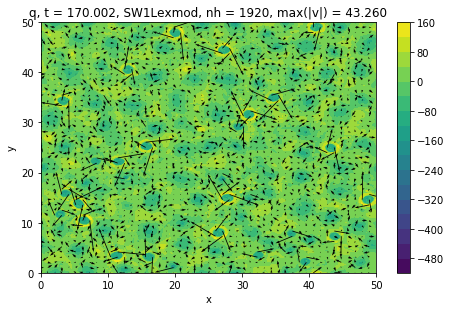# Fluiddyn: open-source, open-science and Python for the fluid dynamics community¶

See https://gfdyn.bitbucket.io for the slides

Ashwin Vishnu Mohanan

Ph.D. student in Geophysical Fluid Dynamics

Linné FLOW Centre, KTH Mechanics

Supervisors: Erik Lindborg, Pierre Augier## A possible scientific utopia¶

• Transparency in scientific methods and results

• Ease of reproducibility

• Openness to full scrutiny

• No more "reinventing the wheel" - particularly in code development

## How do we get there?¶

• Open science: Open-data, Open-source, Open-access, Open-courseware, Open-methodology, Open-peer-review ...

• Reusable code in sciences

• No "code decay": benefit from recent and future software and hardware developments

## The rise of Scientific Python¶

• mature, efficient libraries with huge industry and community support• good coding practices, forges, documentation, unittests, continuous integration, benchmarking

## The rise of Scientific Python¶

• specialized libraries by and for scientific communities (oriented towards subjects)

Only the very beginning of this trend in fluid mechanics!## Why Python?¶

### Pros¶

• Versatile programming language with gentle learning curve

• Easy to migrate. Very similar to MATLAB/Octave syntax

• Interpreted, not compiled language

• Automatic documentation, testing, code-coverage analysis

### Cons¶

• Low performance compared to compiled languages C++, Fortran

## Why Python?¶

We should continue to move to higher levels of abstraction, just like we've done before. We should trade application speed for increased productivity, stability and maintainability. Programmer time is almost always more expensive than CPU time. We aren't writing applications in assembler anymore for the same reason we shouldn't be writing applications in C anymore. - From Haskell wiki

### Example: Moving average filter algorithm - MATLAB vs Python¶

Note: without using any one-line functions

## Why Python?¶

### Moving average filter in MATLAB / Octave¶

In [ ]:
 v = .01; f = 100; fs = 5000; t = 0:1/fs:.03
x = sin(2 * pi * f * t);                             %original signal
r = sqrt(v) * randn(1, length(t));                %noise
Xw = x + r;                                      %signal plus noise (filter input)
y = zeros(___,'like',x)
for n = 3:length(Xw),
y(n) = sum(Xw(n - 2:n)) / 3;       %y[n] is the filtered signal
end
plot(y); hold; plot(x,'r');     %plot the original signal over filtered signal


## Why Python?¶

### Moving average filter in Python¶

In :
 from pylab import *

v = .01; f = 100; fs = 5000; t = arange(0, .03, 1 /fs)
x = sin(2 * pi * f * t)                             # original signal
r = sqrt(v) * random(len(t))                    # noise
Xw = x + r                                      # signal plus noise (filter input)
y = zeros_like(x)
for n in range(3, len(Xw)):
y[n] = sum(Xw[n - 2:n]) / 3       # y[n] is the filtered signal
plot(y); plot(x,'r'); show()   # plot the original signal over filtered signal## Fluid mechanics¶

Science of flows.

### Methods¶

• Experiments
• Analytical computations
• Numerical simulations

• Strong dominance of Fortran and Matlab
• Close-source and old-school open-source software
• Open-science and open-data still weak

## FluidDyn project: a suite of Python packages for fluid dynamics¶

Open-source, documented, tested, continuous integration

• fluiddyn: base package containing utilities
• fluidlab: control of laboratory experiments
• fluidimage: scientific treatments of images (PIV)
• fluidfft: C++ / Python Fourier transform library (highly distributed, MPI, CPU/GPU, 2D and 3D)
• fluidsim: pseudo-spectral simulations in 2D and 3D
• fluidfoam: Python utilities for openfoam

Main developers: Pierre Augier (LEGI), Cyrille Bonamy (LEGI), Antoine Campagne (LEGI), Ashwin Vishnu (KTH), Julien Salort (ENS Lyon).# Numerical simulations¶

## fluidsim: pseudo-spectral simulations in 2D and 3D¶

• solves Burgers, Navier Stokes, Shallow water, Föppl-von Kármán equations using a shared code base
• highly modular (object oriented solvers)
• efficient (Cython, Pythran, mpi4py, h5py)
• on-the-fly data processing
• built-in specialized plotting functions

## fluidsim: Plotting preliminary results¶

• Statistics and useful data are computed and saved on-the-fly

#### Time averaged energy spectra¶

In :
%%capture
import fluidsim as fls


In :
%%capture --no-display
sim.output.spectra.plot2d(tmin=100, delta_t=0, coef_compensate=0.)## fluidsim: Plotting preliminary results¶

#### Pseudo-colour plot of potential vorticity¶

In :
sim.output.phys_fields --no-display
sim.output.physkey_field=elds.plot(key_field='q')## fluidlab: control of experiments in fluid mechanics¶

(Pierre Augier, LEGI & Julien Salors, ENS Lyon)

### Physical experiments can be seen as the interaction of autonomous physical objects¶

• Object-oriented programming
• Very easy to write instrument drivers
• Automatic documentation for the instrument drivers
• Simple servers with the Python package rcpy

#### Example for the carriage¶

• motor.py
• position_sensor.py
• position_sensor_server.py
• position_sensor_client.py
• carriage.py
• carriage_server.py
• carriage_client.py

## The MILESTONE experiment: stratified and rotating turbulence in the Coriolis platform¶

A collaboration between KTH, Stockholm, Sweden and LEGI, University of Grenoble, France#### The Coriolis platform (13 m diameter)

Used by international researchers through European projects (Euhit, Hydralab).

## fluidlab: control of experiments in fluid mechanics¶

A little bit of Graphical User Interface is easy, fun and useful. We use PyQt.Remark: reusable code, here, random movement for another experiment.

## fluidimage: scientific treatments of images¶

(Pierre Augier, Cyrille Bonamy & Antoine Campagne, LEGI; and Ashwin Vishnu, KTH)

Software for images preprocessing and Particle Image Velocimetry (PIV) computation

Many images (~ 20 To of raw data): embarrassingly parallel problem

• Clusters and PC
• CPU/GPU
• Asynchronous computations (topologies of treatments, IO and CPU bounded parts are splitted, compatible with big data frameworks as Storm)
• Efficient algorythms and tools for fast computation with Python (Pythran, Theano, Pycuda, ...)

### Utilities to display and analyze the PIV fields¶

• Plots of PIV fields (similar to PivMat, a Matlab library by F. Moisy)
• Calcul of spectra, anisotropic structure functions, characteristic turbulent length scales

## fluidimage: PIV computation¶

Example of scripts to launch a PIV computation:

from fluidimage.topologies.piv import TopologyPIV

params = TopologyPIV.create_default_params()

params.series.path = '../../image_samples/Karman/Images'
params.series.ind_start = 1
params.piv0.shape_crop_im0 = 32
params.multipass.number = 2
params.multipass.use_tps = True
params.saving.how = 'complete'
params.saving.postfix = 'piv_complete'

topology = TopologyPIV(params, logging_level='info')
topology.compute()


Remark: parameters in an instance of fluiddyn.util.paramcontainer.ParamContainer. Much better than in text files or free Python variables!

• avoid typing errors
• the user can easily look at the available parameters and their default value
• documentation for the parameters (for example for the PIV topology)

## fluidimage: Launching PIV computation on cluster¶

Remark: launching computations on cluster is highly simplified by using fluiddyn:

from fluiddyn.clusters.snic import Beskow

cluster = Beskow()

cluster.submit_script(
'piv_complete.py', name_run='fluidimage',
nb_nodes=1, nb_cores_per_node=8, email='johndoe@kth.se')


Partial conclusion: Open-data, data in auto-descriptive formats (hdf5, netcdf, ...) + code to use and understand the data.

## Conclusions¶

1. Science in fluid mechanics with open-source methods and Python

2. Development of open-source, clean, reusable codes (fluiddyn project)

Issues